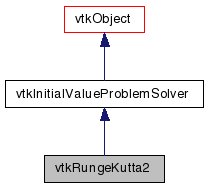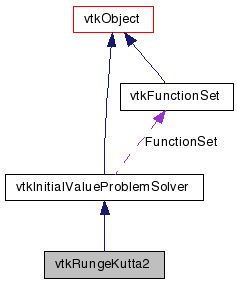# vtkRungeKutta2 Class Reference

`#include <vtkRungeKutta2.h>`

Inheritance diagram for vtkRungeKutta2:[legend]
Collaboration diagram for vtkRungeKutta2:[legend]

## Detailed Description

Integrate an initial value problem using 2nd order Runge-Kutta method.

This is a concrete sub-class of vtkInitialValueProblemSolver. It uses a 2nd order Runge-Kutta method to obtain the values of a set of functions at the next time step.

vtkInitialValueProblemSolver vtkRungeKutta4 vtkRungeKutta45 vtkFunctionSet

Definition at line 33 of file vtkRungeKutta2.h.

## Public Types

typedef
vtkInitialValueProblemSolver
Superclass

## Public Member Functions

virtual const char * GetClassName ()
virtual int IsA (const char *type)
virtual int ComputeNextStep (double *xprev, double *xnext, double t, double &delT, double maxError, double &error)
virtual int ComputeNextStep (double *xprev, double *dxprev, double *xnext, double t, double &delT, double maxError, double &error)
virtual int ComputeNextStep (double *xprev, double *xnext, double t, double &delT, double &delTActual, double minStep, double maxStep, double maxError, double &error)
virtual int ComputeNextStep (double *xprev, double *dxprev, double *xnext, double t, double &delT, double &delTActual, double minStep, double maxStep, double maxError, double &error)

## Static Public Member Functions

static int IsTypeOf (const char *type)
static vtkRungeKutta2SafeDownCast (vtkObject *o)
static vtkRungeKutta2New ()

## Protected Member Functions

vtkRungeKutta2 ()
~vtkRungeKutta2 ()

## Member Typedef Documentation

Reimplemented from vtkInitialValueProblemSolver.

Definition at line 36 of file vtkRungeKutta2.h.

## Constructor & Destructor Documentation

 vtkRungeKutta2::vtkRungeKutta2 ( ) ` [protected]`

 vtkRungeKutta2::~vtkRungeKutta2 ( ) ` [protected]`

## Member Function Documentation

 virtual const char* vtkRungeKutta2::GetClassName ( ) ` [virtual]`

Reimplemented from vtkInitialValueProblemSolver.

 static int vtkRungeKutta2::IsTypeOf ( const char * name ) ` [static]`

Return 1 if this class type is the same type of (or a subclass of) the named class. Returns 0 otherwise. This method works in combination with vtkTypeMacro found in vtkSetGet.h.

Reimplemented from vtkInitialValueProblemSolver.

 virtual int vtkRungeKutta2::IsA ( const char * name ) ` [virtual]`

Return 1 if this class is the same type of (or a subclass of) the named class. Returns 0 otherwise. This method works in combination with vtkTypeMacro found in vtkSetGet.h.

Reimplemented from vtkInitialValueProblemSolver.

 static vtkRungeKutta2* vtkRungeKutta2::SafeDownCast ( vtkObject * o ) ` [static]`

Reimplemented from vtkInitialValueProblemSolver.

 static vtkRungeKutta2* vtkRungeKutta2::New ( ) ` [static]`

Construct a vtkRungeKutta2 with no initial FunctionSet.

Reimplemented from vtkObject.

 virtual int vtkRungeKutta2::ComputeNextStep ( double * xprev, double * xnext, double t, double & delT, double maxError, double & error ) ` [inline, virtual]`

Given initial values, xprev , initial time, t and a requested time interval, delT calculate values of x at t+delT (xnext). delTActual is always equal to delT. Since this class can not provide an estimate for the error error is set to 0. maxStep, minStep and maxError are unused. This method returns an error code representing the nature of the failure: OutOfDomain = 1, NotInitialized = 2, UnexpectedValue = 3

Reimplemented from vtkInitialValueProblemSolver.

Definition at line 48 of file vtkRungeKutta2.h.

 virtual int vtkRungeKutta2::ComputeNextStep ( double * xprev, double * dxprev, double * xnext, double t, double & delT, double maxError, double & error ) ` [inline, virtual]`

Given initial values, xprev , initial time, t and a requested time interval, delT calculate values of x at t+delT (xnext). delTActual is always equal to delT. Since this class can not provide an estimate for the error error is set to 0. maxStep, minStep and maxError are unused. This method returns an error code representing the nature of the failure: OutOfDomain = 1, NotInitialized = 2, UnexpectedValue = 3

Reimplemented from vtkInitialValueProblemSolver.

Definition at line 57 of file vtkRungeKutta2.h.

 virtual int vtkRungeKutta2::ComputeNextStep ( double * xprev, double * xnext, double t, double & delT, double & delTActual, double minStep, double maxStep, double maxError, double & error ) ` [inline, virtual]`

Given initial values, xprev , initial time, t and a requested time interval, delT calculate values of x at t+delT (xnext). delTActual is always equal to delT. Since this class can not provide an estimate for the error error is set to 0. maxStep, minStep and maxError are unused. This method returns an error code representing the nature of the failure: OutOfDomain = 1, NotInitialized = 2, UnexpectedValue = 3

Reimplemented from vtkInitialValueProblemSolver.

Definition at line 67 of file vtkRungeKutta2.h.

 virtual int vtkRungeKutta2::ComputeNextStep ( double * xprev, double * dxprev, double * xnext, double t, double & delT, double & delTActual, double minStep, double maxStep, double maxError, double & error ) ` [virtual]`

Given initial values, xprev , initial time, t and a requested time interval, delT calculate values of x at t+delT (xnext). delTActual is always equal to delT. Since this class can not provide an estimate for the error error is set to 0. maxStep, minStep and maxError are unused. This method returns an error code representing the nature of the failure: OutOfDomain = 1, NotInitialized = 2, UnexpectedValue = 3

Implements vtkInitialValueProblemSolver.

The documentation for this class was generated from the following file:

Generated on Wed Aug 24 12:04:34 2011 for VTK by1.5.6